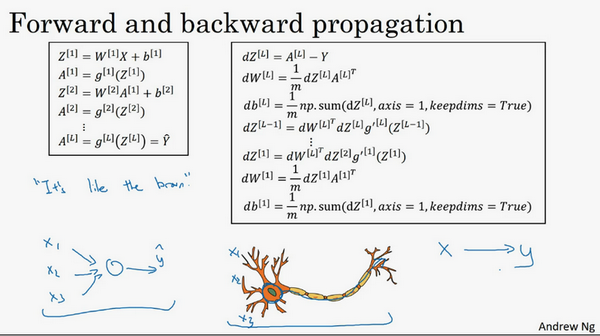## 第四周：深层神经网络(Deep Neural Networks)

### 4.1 深层神经网络（Deep L-layer neural network）

1.逻辑回归，结构如下图左边。一个隐藏层的神经网络，结构下图右边：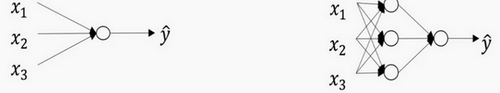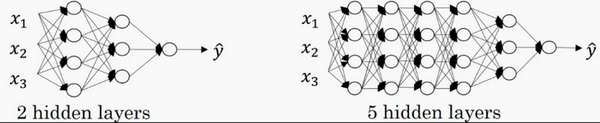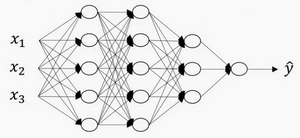### 4.2 前向传播和反向传播（Forward and backward propagation）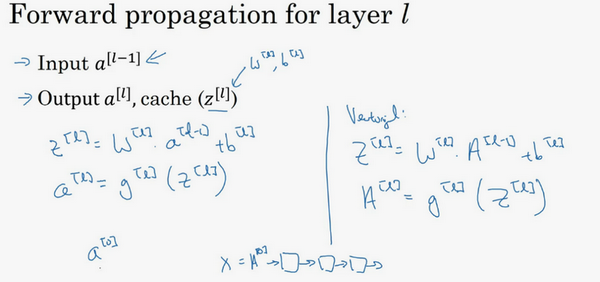${{a}^{[l]}}={{g}^{[l]}}\left( {{z}^{[l]}}\right)$

${A}^{[l]}={g}^{[l]}({Z}^{[l]})$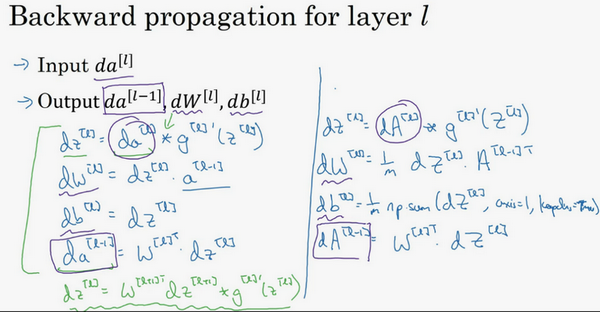（1）$d{{z}^{[l]}}=d{{a}^{[l]}}*{{g}^{[l]}}'( {{z}^{[l]}})$

（2）$d{{w}^{[l]}}=d{{z}^{[l]}}\cdot{{a}^{[l-1]}}~$

（3）$d{{b}^{[l]}}=d{{z}^{[l]}}~~$

（4）$d{{a}^{[l-1]}}={{w}^{\left[ l \right]T}}\cdot {{dz}^{[l]}}$

（5）$d{{z}^{[l]}}={{w}^{[l+1]T}}d{{z}^{[l+1]}}\cdot \text{ }{{g}^{[l]}}'( {{z}^{[l]}})~$

（6）$d{{Z}^{[l]}}=d{{A}^{[l]}}*{{g}^{\left[ l \right]}}'\left({{Z}^{[l]}} \right)~~$

（7）$d{{W}^{[l]}}=\frac{1}{m}\text{}d{{Z}^{[l]}}\cdot {{A}^{\left[ l-1 \right]T}}$

（8）$d{{b}^{[l]}}=\frac{1}{m}\text{ }np.sum(d{{z}^{[l]}},axis=1,keepdims=True)$

（9）$d{{A}^{[l-1]}}={{W}^{\left[ l \right]T}}.d{{Z}^{[l]}}$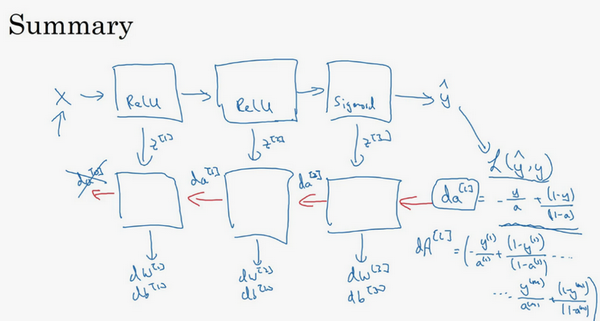### 4.3 深层网络中的前向传播（Forward propagation in a Deep Network）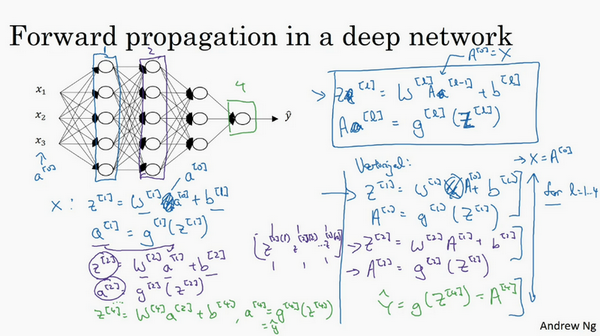${{Z}^{[l]}}={{W}^{[l]}}{{a}^{[l-1]}}+{{b}^{[l]}}$${{A}^{[l]}}={{g}^{[l]}}{({Z}^{[l]})}$ (${{A}^{}} = X)$

### 4.4 核对矩阵的维数（Getting your matrix dimensions right）

$w$的维度是（下一层的维数，前一层的维数），即${{w}^{[l]}}$: (${{n}^{[l]}}$,${{n}^{[l-1]}}$)；

$b$的维度是（下一层的维数，1），即:

${{b}^{[l]}}$ : (${{n}^{[l]}},1)$

${{z}^{[l]}}$,${{a}^{[l]}}$: $({{n}^{[l]}},1)$;

${{dw}^{[l]}}$${{w}^{[l]}}$维度相同，${{db}^{[l]}}$${{b}^{[l]}}$维度相同，且$w$$b$向量化维度不变，但$z$,$a$以及$x$的维度会向量化后发生变化。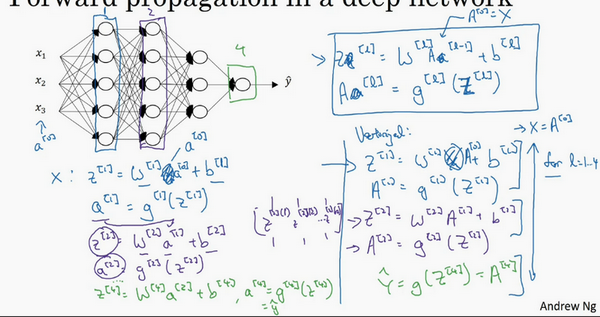${Z}^{[l]}$可以看成由每一个单独的${Z}^{[l]}$叠加而得到，${Z}^{[l]}=({{z}^{[l]}}，{{z}^{[l]}}，{{z}^{[l]}}，…，{{z}^{[l][m]}})$

$m$为训练集大小，所以${Z}^{[l]}$的维度不再是$({{n}^{[l]}},1)$，而是$({{n}^{[l]}},m)$

${A}^{[l]}$$({n}^{[l]},m)$${A}^{} = X =({n}^{[l]},m)$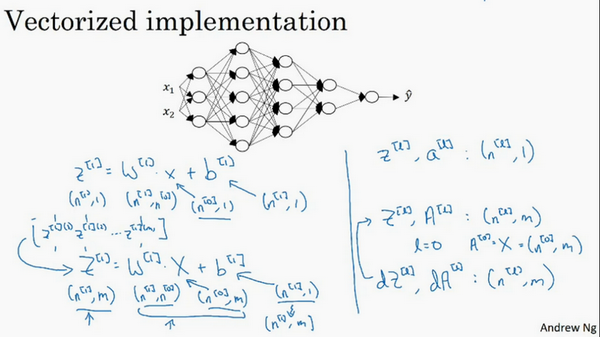### 4.5 为什么使用深层表示？（Why deep representations?）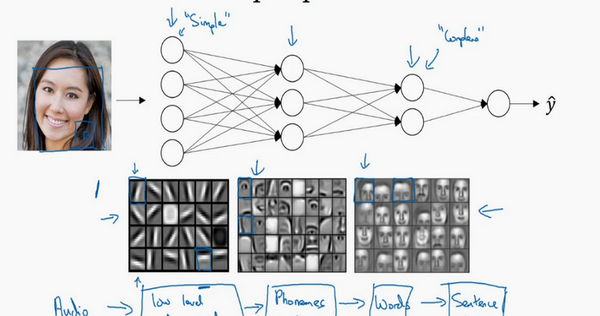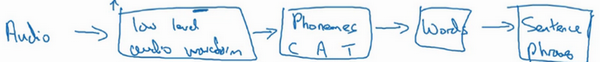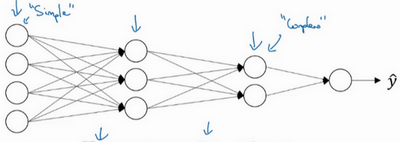Small：隐藏单元的数量相对较少

Deep：隐藏层数目比较多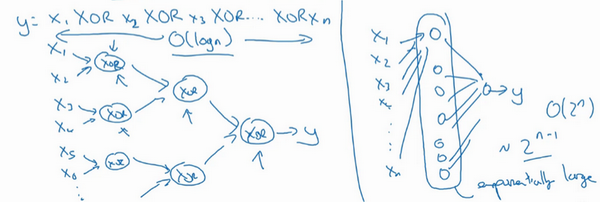### 4.6 搭建神经网络块（Building blocks of deep neural networks）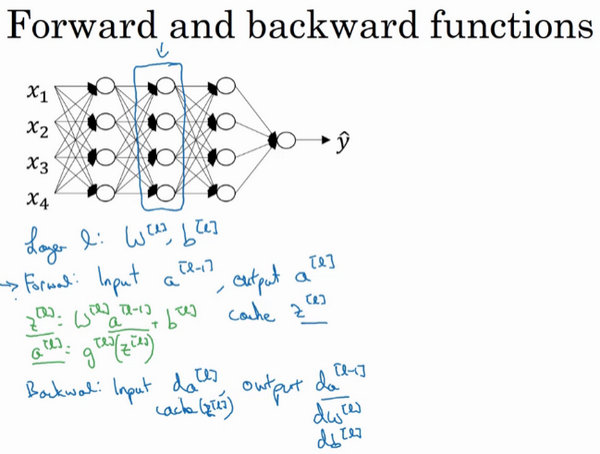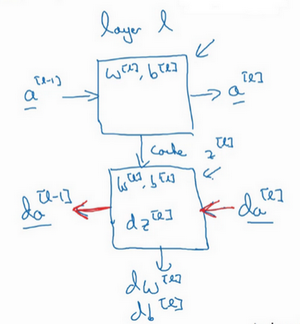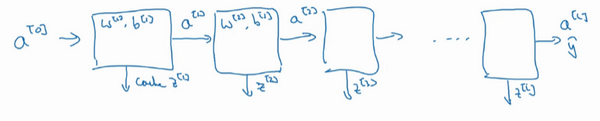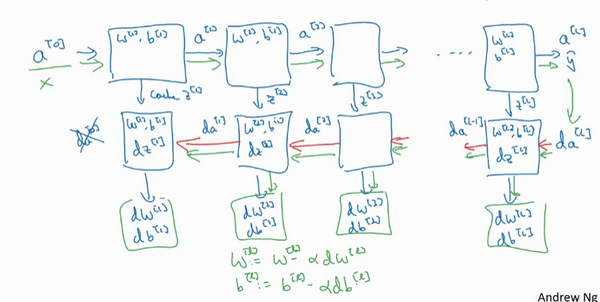### 4.7 参数VS超参数（Parameters vs Hyperparameters）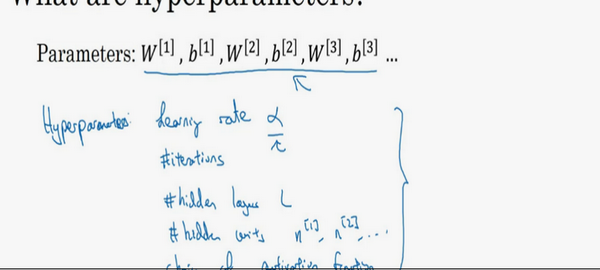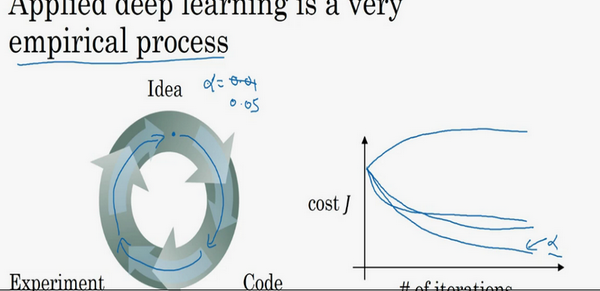Idea—Code—Experiment—Idea这个循环，尝试各种不同的参数，实现模型并观察是否成功，然后再迭代。

### 4.8 深度学习和大脑的关联性（What does this have to do with the brain?）